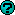View Single Post
 djfranknittiRegistered User Join Date: Oct 2004 Location: , , . Posts: 3 Thanks: 0 Thanked 0 Times in 0 Postscreating a bulletin board system

hi, my name is frank ivey and i am a avid reader of books from he wrox publishing. I am also a big fan of php and mysql. I just bought the book "beginning php, apache, mysql web development" and I must say that it has bee very edcational and easy to read. I am currently talcking a problem in chapter 15, creating a BB system. the giving code for http.php is suppose to redirect the user to index.php. but i think it is trying to redirect to itself instead (http.php).

when ever i try to submit a login it calls the transact-user.php script. This script works find until it calls the redirct function from http.php. Then I get an error that states "Could not redirect; Headers already sent (output)." which is the error i told it to print if it cant find the header.

I am posting my transact-user.php code and http.php code at the bottom. This code is the same as it is in the book.

transact-user.php:

<?php

require_once 'conn.php';
require_once 'http.php';

if (isset(\$_REQUEST['action'])) {
switch (\$_REQUEST['action']) {
if (isset(\$_POST['email'])
and isset(\$_POST['passwd']))
{
\$sql = "SELECT id,access_lvl,name,last_login " .
"FROM forum_users " .
"WHERE email='" . \$_POST['email'] . "' " .
"AND passwd='" . \$_POST['passwd'] . "'";
\$result = mysql_query(\$sql,\$conn)
or die('Could not look up user information; ' . mysql_error());

if (\$row = mysql_fetch_array(\$result)) {
session_start();
\$_SESSION['user_id'] = \$row['id'];
\$_SESSION['access_lvl'] = \$row['access_lvl'];
\$_SESSION['name'] = \$row['name'];
\$sql = "UPDATE forum_users SET last_login = '".
date("Y-m-d H:i:s",time()) . "' ".
"WHERE id = ". \$row['id'];
mysql_query(\$sql,\$conn)
or die(mysql_error()."<br>".\$sql);
}
}
redirect('index.php');
break;

case 'Logout':
session_start();
session_unset();
session_destroy();

redirect('index.php');
break;

case 'Create Account':
if (isset(\$_POST['name'])
and isset(\$_POST['email'])
and isset(\$_POST['passwd'])
and isset(\$_POST['passwd2'])
and \$_POST['passwd'] == \$_POST['passwd2'])
{
\$sql = "INSERT INTO forum_users ".
"VALUES ('" . \$_POST['email'] . "','" .
\$_POST['name'] . "','" . \$_POST['passwd'] . "','".
date("Y-m-d H:i:s",time()). "','".
date("Y-m-d H:i:s",time()). "')";

mysql_query(\$sql,\$conn)
or die('Could not create user account; ' . mysql_error());

session_start();
\$_SESSION['user_id'] = mysql_insert_id(\$conn);
\$_SESSION['access_lvl'] = 1;
\$_SESSION['name'] = \$_POST['name'];
}
redirect('index.php');
break;

case 'Modify Account':
if (isset(\$_POST['name'])
and isset(\$_POST['email'])
and isset(\$_POST['accesslvl'])
and isset(\$_POST['userid']))
{
\$sql = "UPDATE forum_users " .
"SET email='" . \$_POST['email'] .
"', name='" . \$_POST['name'] .
"', access_lvl=" . \$_POST['accesslvl'] .
", signature='" . \$_POST['signature'] . "' " .
" WHERE id=" . \$_POST['userid'];

mysql_query(\$sql,\$conn)
or die('Could not update user account... ' . mysql_error() .
'<br>SQL: ' . \$sql);
}
break;

case 'Edit Account':
if (isset(\$_POST['name'])
and isset(\$_POST['email'])
and isset(\$_POST['accesslvl'])
and isset(\$_POST['userid']))
{
\$chg_pw=FALSE;
if (isset(\$_POST['oldpasswd'])
and \$_POST['oldpasswd'] != '') {
\$sql = "SELECT passwd FROM forum_users " .
"WHERE id=" . \$_POST['userid'];
\$result = mysql_query(\$sql) or die(mysql_error());
if (\$row = mysql_fetch_array(\$result)) {
if ((\$row['passwd'] == \$_POST['oldpasswd'])
and (isset(\$_POST['passwd']))
and (isset(\$_POST['passwd2']))
and (\$_POST['passwd'] == \$_POST['passwd2']))
{
\$chg_pw = TRUE;
} else {
redirect('useraccount.php?error=nopassedit');
break;
}
}
}
\$sql = "UPDATE forum_users " .
"SET email='" . \$_POST['email'] .
"', name='" . \$_POST['name'] .
"', access_lvl=" . \$_POST['accesslvl'] .
", signature='" . \$_POST['signature'];
if (\$chg_pw) {
\$sql .= "', passwd='" . \$_POST['passwd'];
}
\$sql .= "' WHERE id=" . \$_POST['userid'];
mysql_query(\$sql,\$conn)
or die('Could not update user account... ' . mysql_error() .
'<br>SQL: ' . \$sql);
}
redirect('useraccount.php?blah=' . \$_POST['userid']);
break;

case 'Send my reminder!':
if (isset(\$_POST['email'])) {
\$sql = "SELECT passwd FROM forum_users " .
"WHERE email='" . \$_POST['email'] . "'";

\$result = mysql_query(\$sql,\$conn)
or die('Could not look up password; ' . mysql_error());

if (mysql_num_rows(\$result)) {
\$row = mysql_fetch_array(\$result);

\$subject = 'Comic site password reminder';
"Comic Book Appreciation site is: " . \$row['passwd'] .
\$_SERVER['HTTP_HOST'] .
\$_POST['email'];

or die('Could not send reminder email.');
}
}
break;
}
}
?>

http.php:
<?php
function redirect(\$url) {
:(:(:(